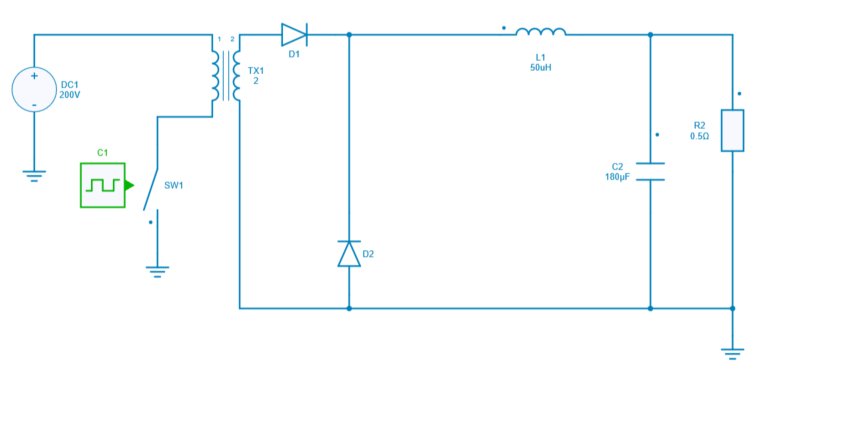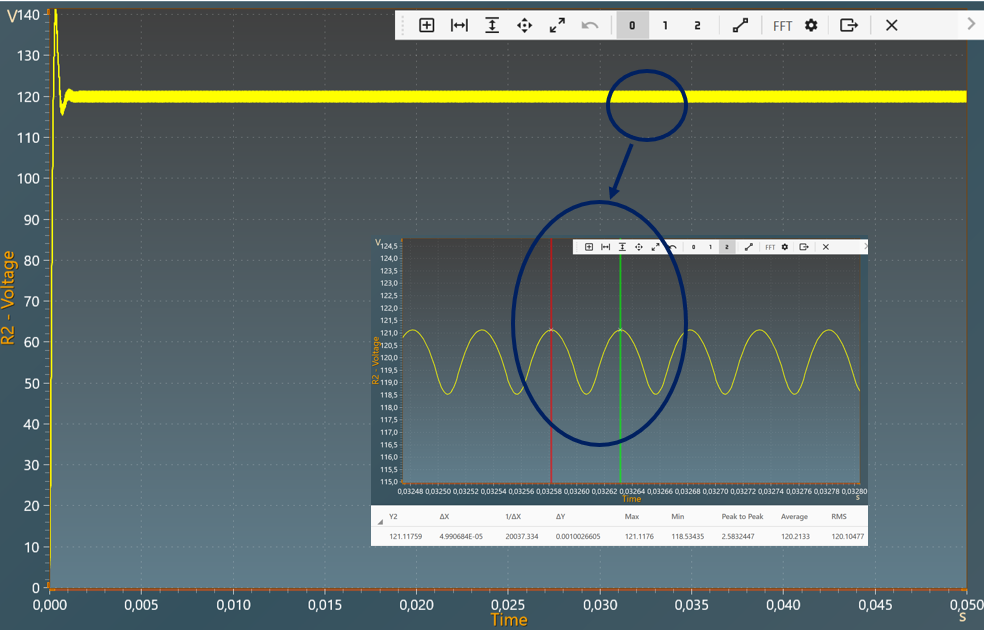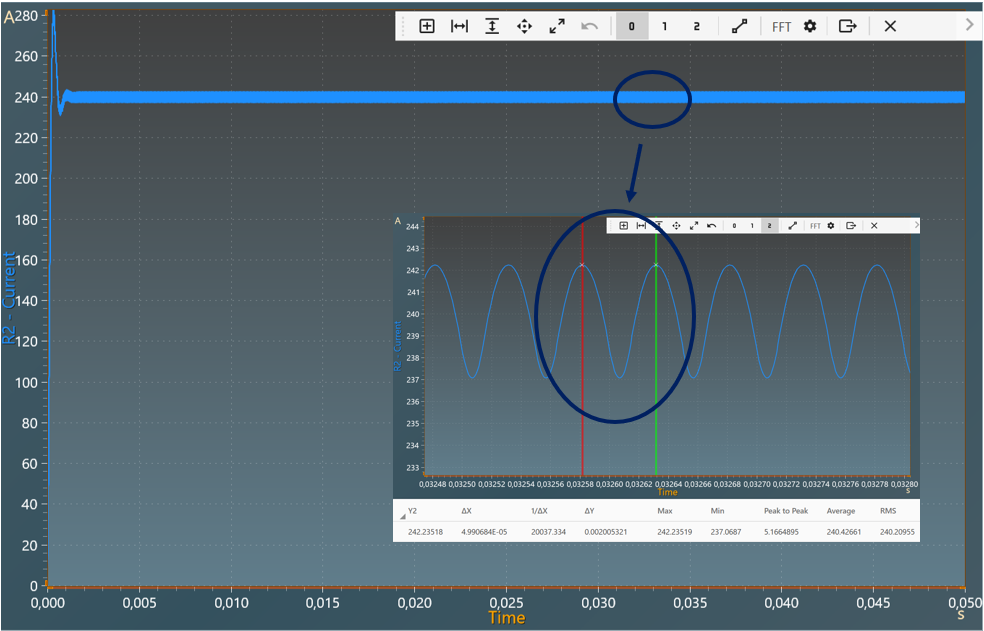Resources

# DC-DC Forward converter

This example shows a DC-DC Forward Converter with:

• an input voltage of 200 V,
• an output voltage of 120 V,
• a power of 28.8 kW transferred at the output resistor.

The Forward converter is a switching power supply circuit that transfers the energy from the primary to the secondary while the switching element is “on,” which is the opposite of a flyback converter. Forward is commonly used used to either increase or decrease DC voltages depending upon the duty cycle value and provide galvanic isolation for the load. Energy is passed directly through the transformer during the transistor’s conduction phase. The output voltage is determined by the input voltage, the transformer turns ratio and the duty cycle.

## Model

In this model, all components are ideal.When the switch is closed, there is the same polarity on transformer circuit diagram so that the diode D1 is conducting. In accordance with the function of the transformer the current transformed from the secondary side flows on the primary side and the linearly increasing magnetizing current. The transformed input voltage is applied to the secondary winding. This serves to charge the choke (inductor). A linearly increasing current flows through the choke and the load.

Now If the switch is opened, the current cannot continue to flow on the primary side. The polarity in the magnetic components reverses so that D1 is blocked on the secondary side. The choke L1 now transfers the stored energy to the load via D2. The current through the choke again decreases linearly.

The core of the transformer has been magnetized by the magnetization current. The remanence of the core material would cause the core to saturate within a few switching cycles. So it has to be demagnetized after each switching period.

## Switch control

The switch model can be directly connected with a control signal. For this control, we use a PWM block with an amplitude of 1, a duty cycle of 0.3 and a frequency of 20KHz.

Here are the values of the other components :

• inductor L1 : 50 µH and initial current = 0A,
• capacitor C2: 200 µF and initial voltage = 0V,
• resistor R2: 0.5 $$\Omega$$,
• diode D1: Rd = 0 $$\Omega$$ and Forward voltage = 0V,
• diode D2: Rd = 0 $$\Omega$$ and Forward voltage = 0V,
• transformer TX1: ratio $$\frac{V2}{V1} =2$$.

## Simulation

The results below show the output voltage across R2 with a duty cycle= 0.3. A zoom shows a measure of the average value of this voltage : 120 V.The results below show the output current through R2 with a duty cycle= 0.3. A zoom shows a measure of the average value of this current : 240 A.The results follow the equation: $$V_{out} =\frac{N_2}{N_1} \times V_{in} \times D$$

with:

• D: Duty cycle
• $$V_{in}$$: Input voltage
• $$V_{out}$$: Output voltage
• N2: Number of turns at secondary
• N1: Number of turns at primary